# Table Q4.1 shows the state transition table for a finite state machine (FSM) with one input...

Table Q4.1 shows the state transition table for a finite state machine (FSM) with one input x, one output z and eight states.

(a) Copy the table of Table Q4.2 into your examination book and determine the states and outputs for the input listed, assuming a start current state of ‘1’. Determine what function the FSM is performing.

(b) Using the implication chart method, determine the minimal number of states. Show clearly your analysis.

(c) Draw the reduced state transition table.

(d) Using a straightforward data assignment for the reduced state transition table, determine the ROM contents for the circuit in Figure Q4.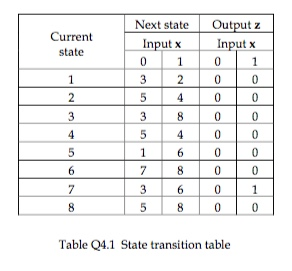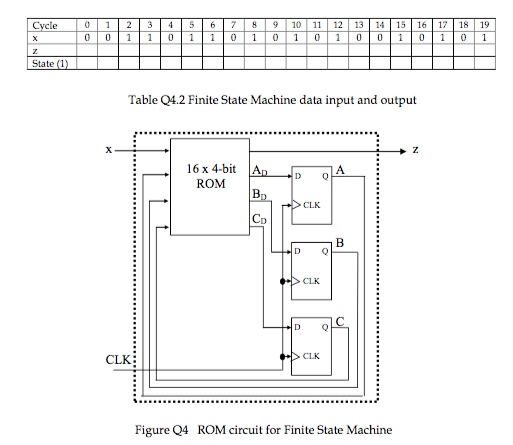Current state Next state Input x Output z Input x 0 800 Table 24.1 State transition table
Cycle 9 10 11 12 13 14 State (1) Table 04.2 Finite State Machine data input and output 16 x 4-bit ROM ADA >CLK > CLK - CLK Figure Q4 ROM circuit for Finite State Machine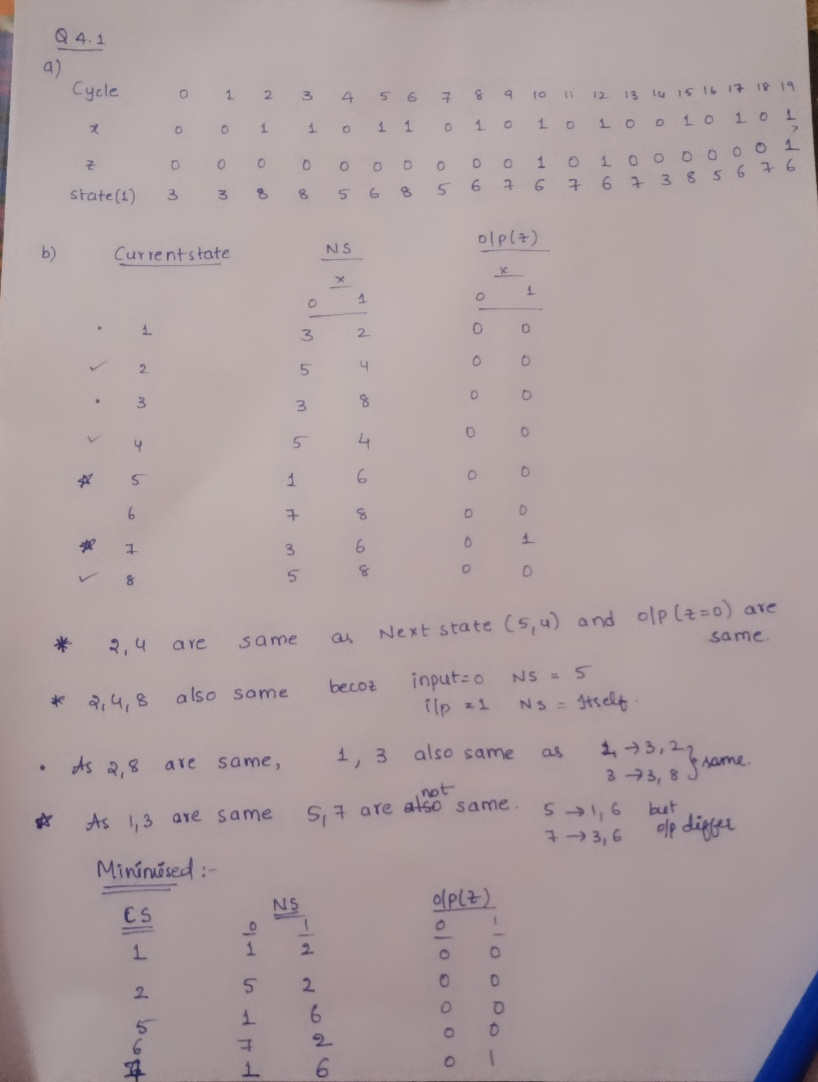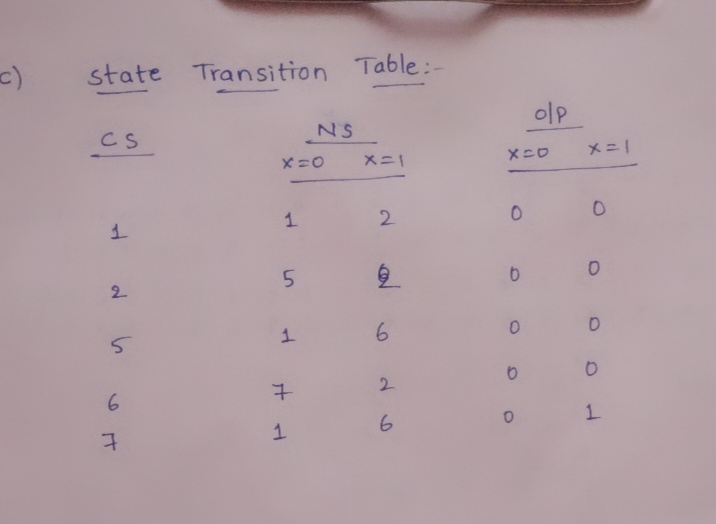##### Add Answer of: Table Q4.1 shows the state transition table for a finite state machine (FSM) with one input...
Similar Homework Help Questions
• ### Question 9 [7 Marks] A state table for a finite state machine (FSM) is given below....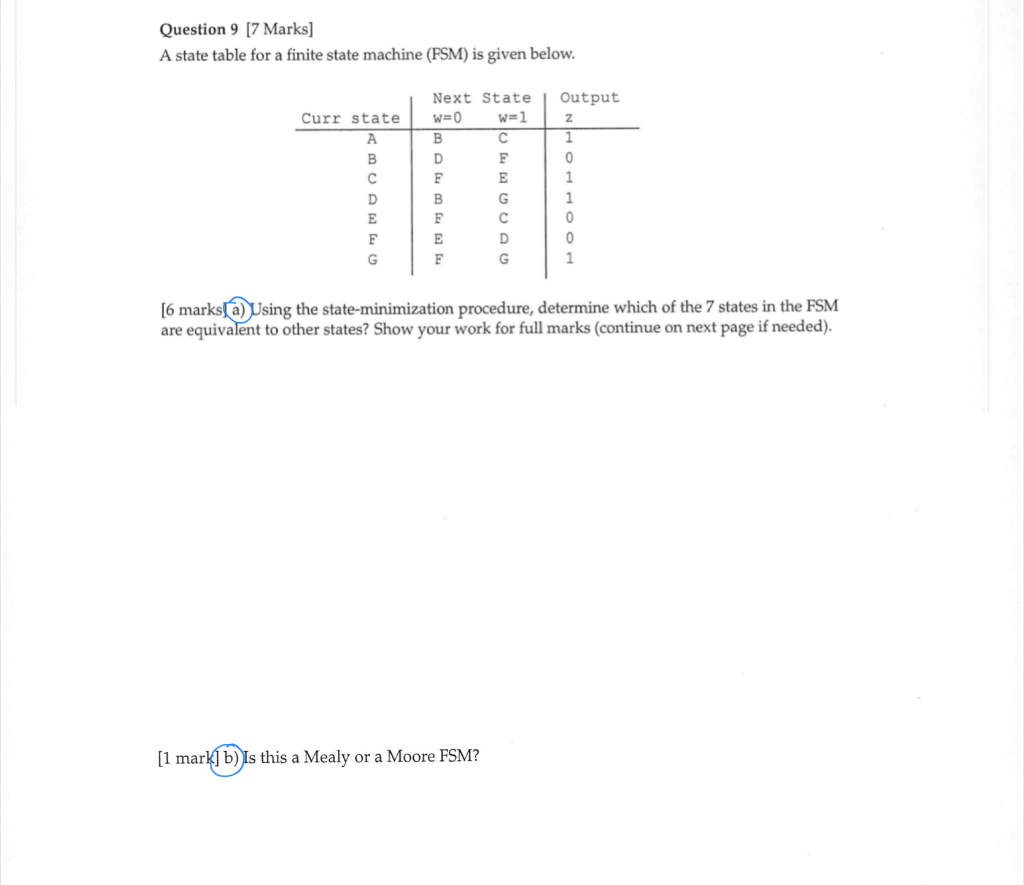Question 9 [7 Marks] A state table for a finite state machine (FSM) is given below. Output Next State w=0 w=1 Curr state 1 [6 marks[a) Using the state-minimization procedure, determine which of the 7 states in the FSM are equivalent to other states? Show your work for full marks (continue on next page if needed). [1 mark] b) Is this a Mealy or a Moore FSM?

• ### a) A synchronous finite state machine (FSM) is described by the state table in Fig. 3. Show how redundant states may...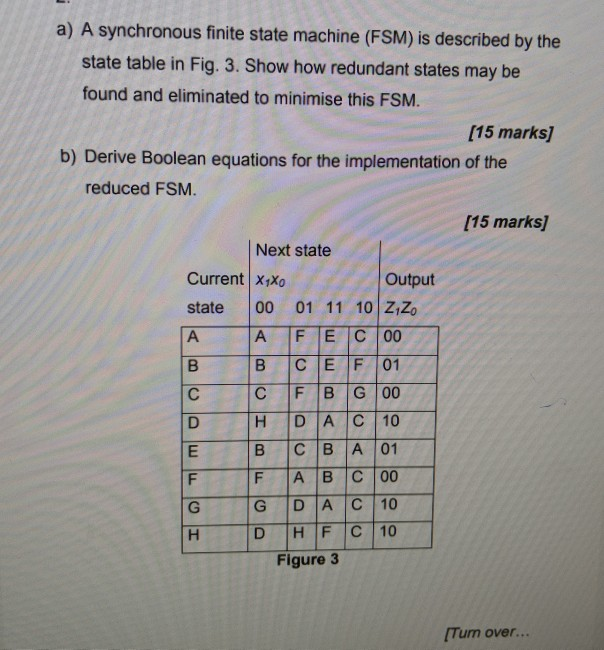a) A synchronous finite state machine (FSM) is described by the state table in Fig. 3. Show how redundant states may be found and eliminated to minimise this FSM. [15 marks) b) Derive Boolean equations for the implementation of the reduced FSM. (15 marks] Next state Output Current X1Xo state 00 01 11 10 Z1Zo A A F E C 00 B C B A 01 F A B C 00 G DİACİ 10 Figure 3 Tum over... a) A...

• ### 0/3 D6.15 Write an assembly main program that implements this Mealy finite state machine. happy The FSM state graph...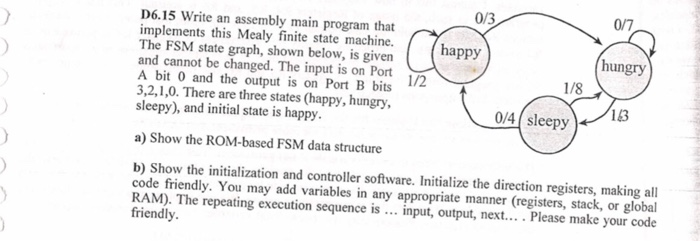0/3 D6.15 Write an assembly main program that implements this Mealy finite state machine. happy The FSM state graph, shown below, is givenP and cannot be changed. The input is on Port A bit 0 and the output is on Port B bits 3,2,1,0. There are three states (happy, hungry, sleepy), and initial state is happy. hungry 1/8 1/2 143 0/4 sleepy a) Show the ROM-based FSM data structure b) Show the initialization and controller software. Initialize the direction registers,...

• ### Consider the following FSM state transition diagram: 7. Let's see if there is an equivalent state machine with fewe...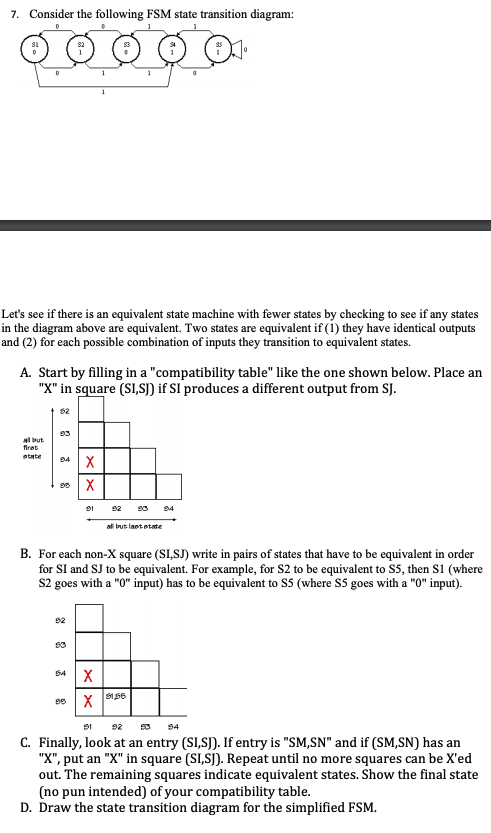Consider the following FSM state transition diagram: 7. Let's see if there is an equivalent state machine with fewer states by checking to see if any states in the diagram above are equivalent. Two states are equivalent if (1) they have identical outputs and (2) for each possible combination of inputs they transition to equivalent states. A. Start by filling in a "compatibility table" like the one shown below. Place an "X" in square (SISI) if SI produces a different...

• ### Option 3 was incorrect. QUESTION 4 Suppose the same finite state machine was re-implemented with the...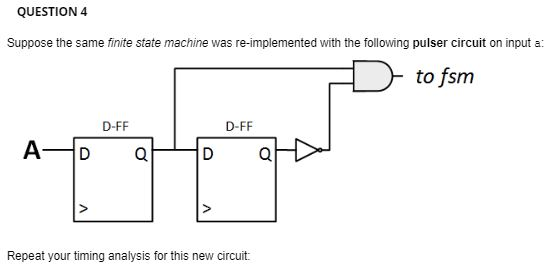Option 3 was incorrect. QUESTION 4 Suppose the same finite state machine was re-implemented with the following pulser circuit on input a: to fsm D-FF D-FF A- D D Repeat your timing analysis for this new circuit: clk a F clk 0 a F clk 0 a F

• ### QUESTION 1 The following finite state machine is designed to produce an output which toggles continuously...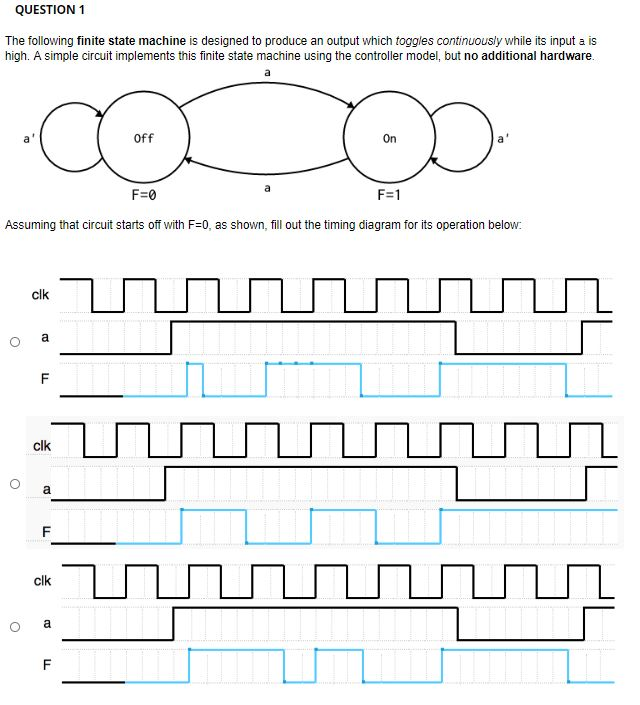QUESTION 1 The following finite state machine is designed to produce an output which toggles continuously while its input a is high. A simple circuit implements this finite state machine using the controller model, but no additional hardware. a Off On F=0 F=1 Assuming that circuit starts off with F=0, as shown, fill out the timing diagram for its operation below: clk a O F clk a F clk O a F QUESTION 2 Take a moment to consider the...

• ### 2. (20 pts.) Write the finite state machine (FSM) of the circuit shown below. Hint: In the given DEMUX below, S2 is...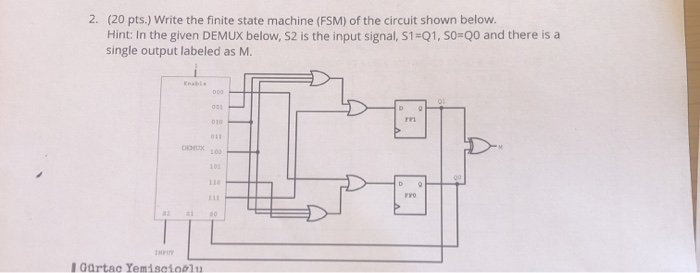2. (20 pts.) Write the finite state machine (FSM) of the circuit shown below. Hint: In the given DEMUX below, S2 is the input signal, S1-Q1, s0-Q0 and there is a single output labeled as M. X100 FrO 113 1 NPUT IGartac Yemisc1o01 2. (20 pts.) Write the finite state machine (FSM) of the circuit shown below. Hint: In the given DEMUX below, S2 is the input signal, S1-Q1, s0-Q0 and there is a single output labeled as M. X100...

• ### These two images for one question. 2. The following finite state machine circuit is a sequence detector, where the state is Y2Y,Yo and the output is Z. Determine the sequence that will take the finit...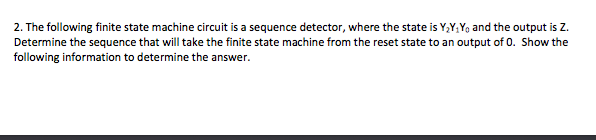These two images for one question. 2. The following finite state machine circuit is a sequence detector, where the state is Y2Y,Yo and the output is Z. Determine the sequence that will take the finite state machine from the reset state to an output of 0. Show the following information to determine the answer. 0 23 CLK a. (10 points) The expression for the inputs to the TFFs. b. (20 points) The state table. c. (15 points) The state diagram....

• ### Derive the state diagram for an FSM that has an input w and an output z. The mach...continues

Derive the state diagram for an FSM that has an input w and an output z. The machine has to generate z = 1 when the previous four values of w were 1001 or 1111;otherwise, z = 0. Overlapping input patterns are allowed. Describe the state assignment and derive a State-assigned Table for the FSM described in problem. Explainwhat the states of the system are and how they relate to the sequence detector problem.

• ### Finite State Machine

(Finite State Machine)a. A finite state recognizer or sequence detector has one input (X) and one output (Z). The output is asserted high (Z=1) whenever the input sequence 1101 has beenobserved during the past four clock cycles, as long as 1110 has never been observed. Draw the state diagram for the finite state recognizer. (Overlapping goodsequences are allowed.)b. Present one possible state assignment for your recognizer of part a.c. A state diagram for a particular finite state machine requires a...

Free Homework App